# 运用代码块的两种方式，让不可能的需求成为可能### 字段默认值

1. 在表单配置界面选中字段
2. 选择函数计算赋默认值
3. 开启自定义函数，使用JavaScript编写代码块

#### 1.身份证号提取信息let birthday = IdCard.substring(6, 10) + “-” + IdCard.substring(10, 12) + “-” + IdCard.substring(12, 14);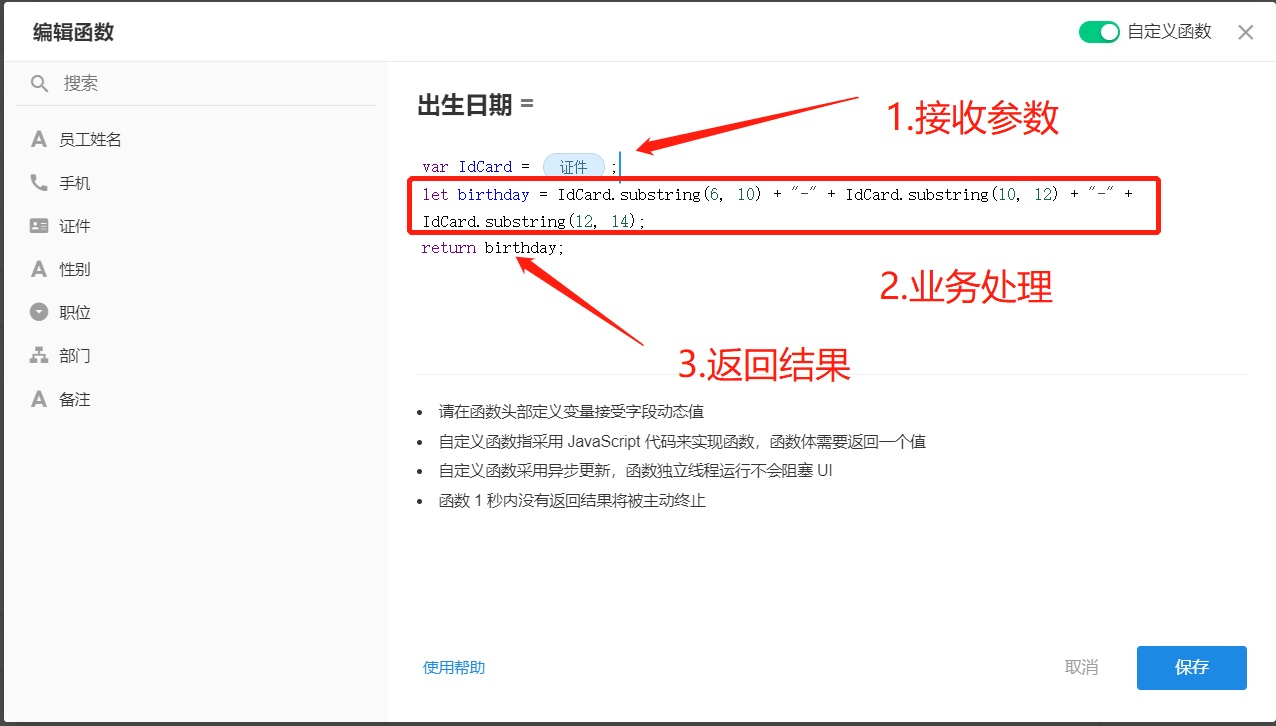if (parseInt(IdCard.substr(16, 1)) % 2 === 1) {

var sex =”男”;

} else {

var sex =”女”;

}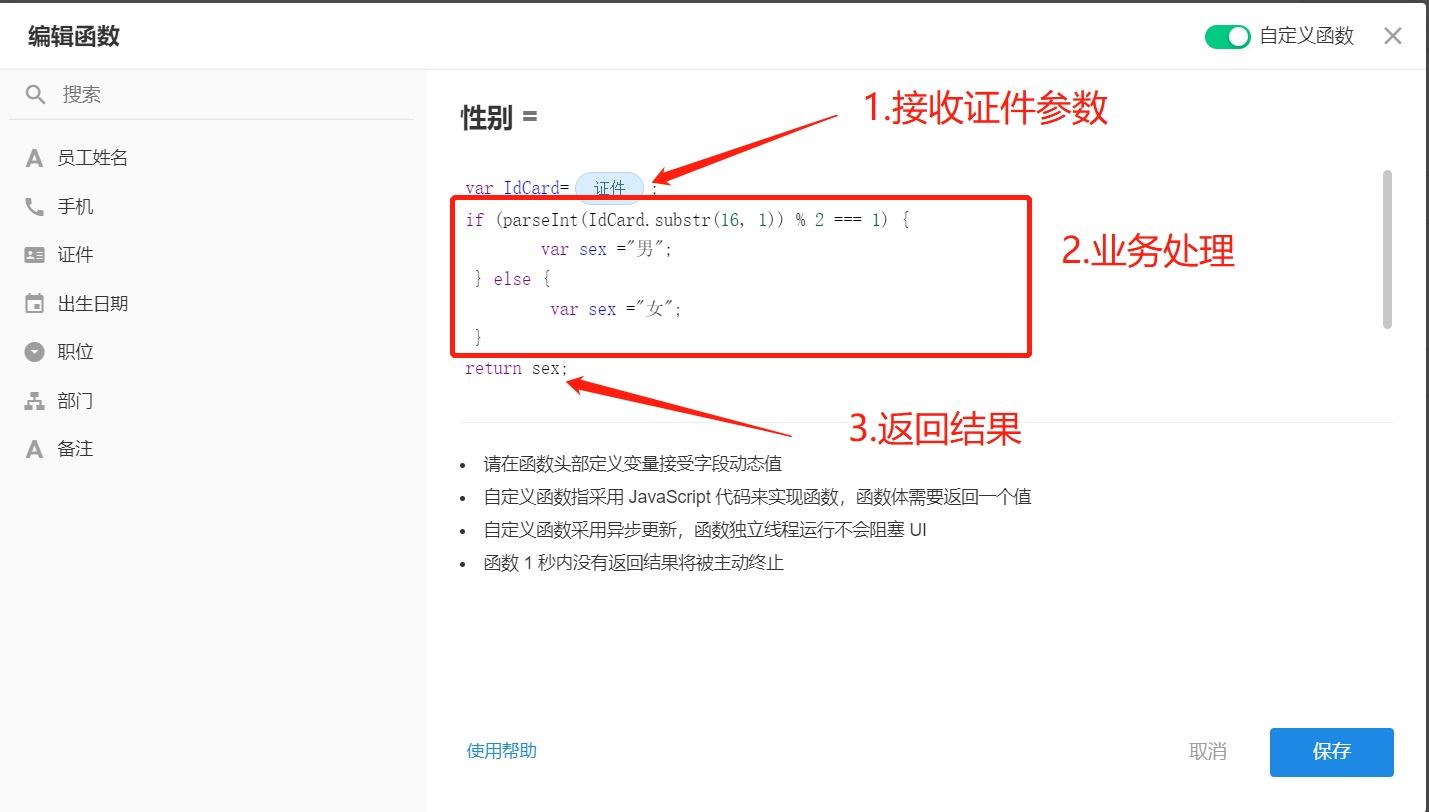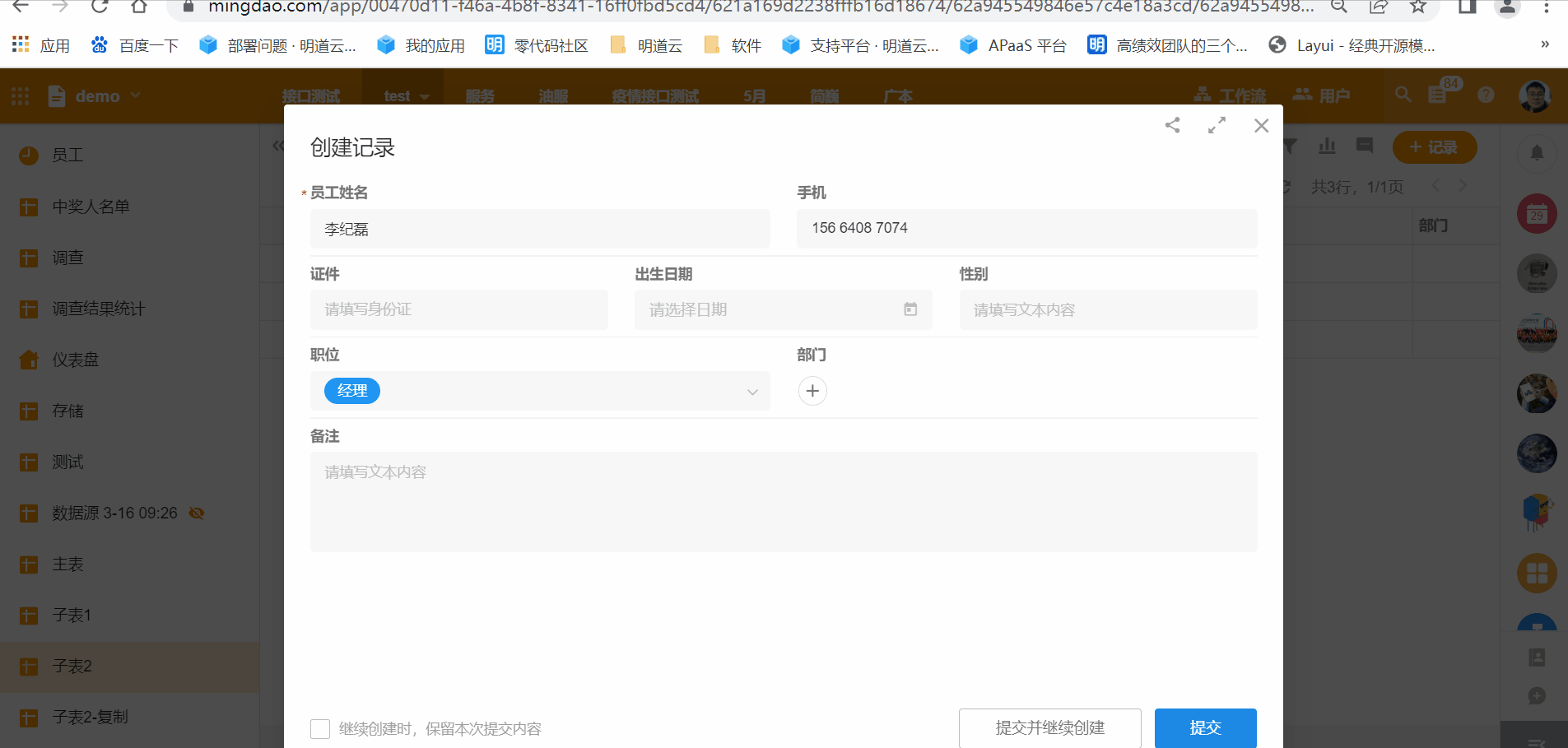#### 2.日期运算

var date = new Date(date);

var date2=new Date(date.getFullYear(), 0, 1);

var day1=date.getDay();

if(day1==0) day1=7;

var day2=date2.getDay();

if(day2==0) day2=7;

d = Math.round((date.getTime() – date2.getTime()+(day2-day1)*(24*60*60*1000)) / 86400000);

var wek=Math.ceil(d /7)+1let d = new Date(time);

var time_stamp=d.valueOf(d)/1000;### 工作流中的复杂逻辑

#### 配置流程

1）确定数据类型2) 编辑代码块

3) 传出数据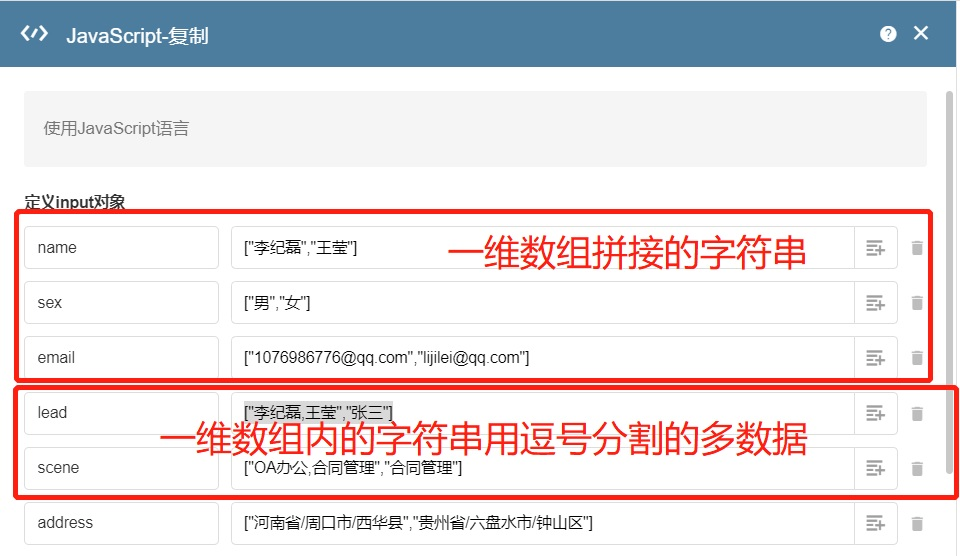4) 测试数据5）将示例数据修改为正式数据，根据需要用在工作流节点中。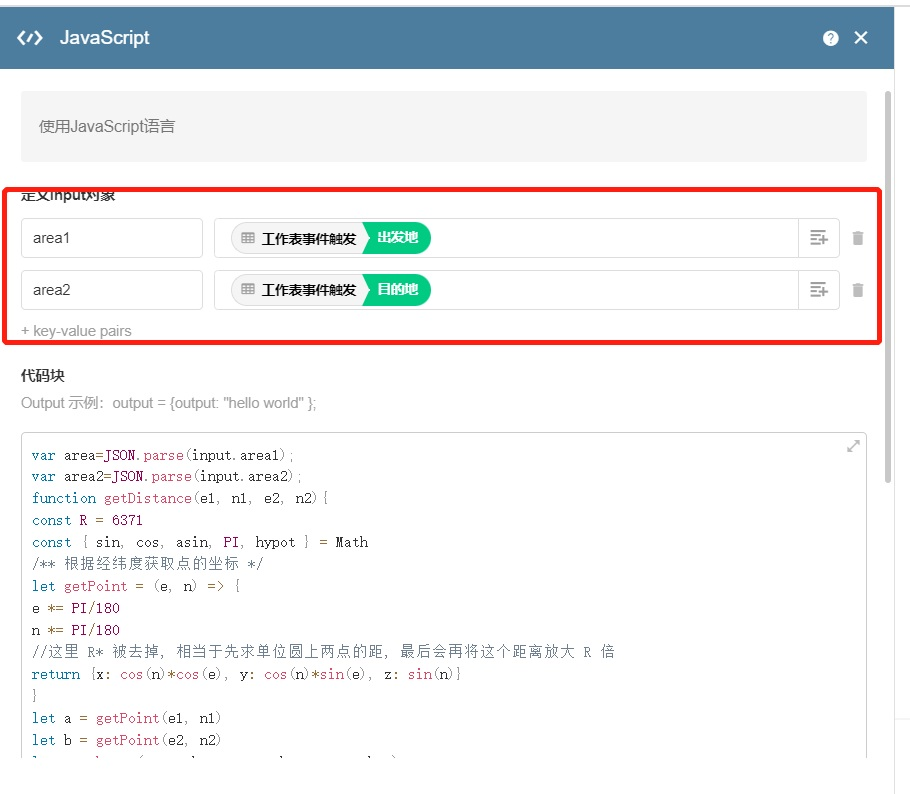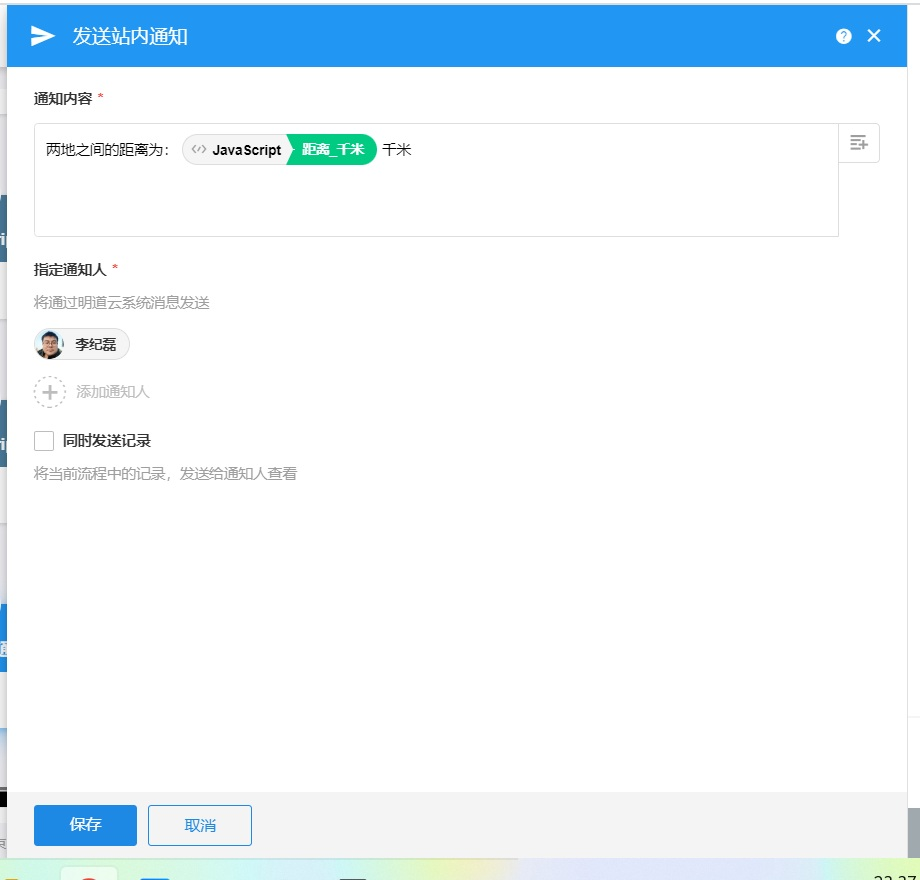#### 六个典型实用的代码块

1.数值计算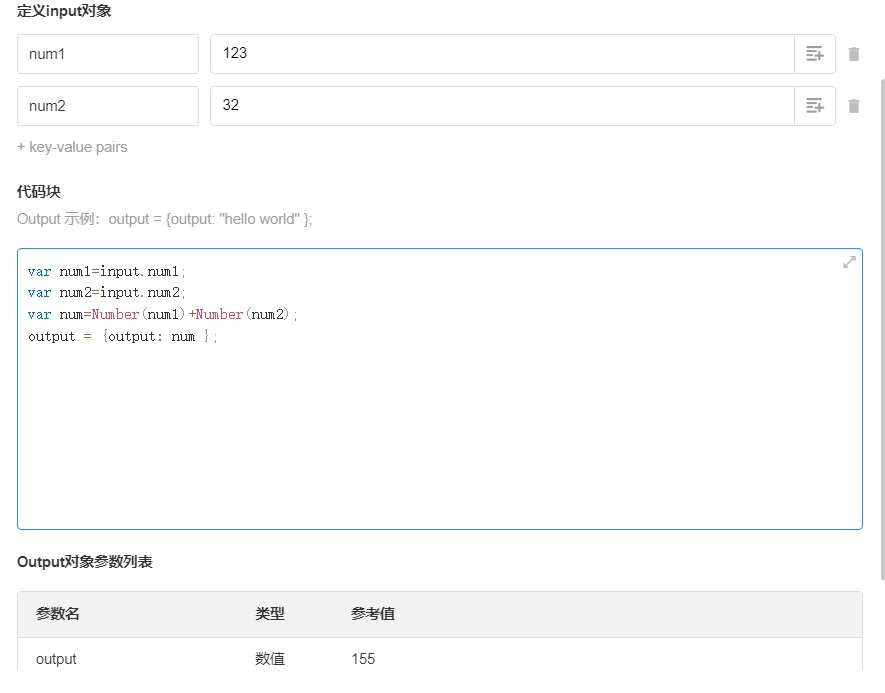var num1=input.num1;

var num2=input.num2;

var num=Number(num1)+Number(num2);

output = {output: num };

2.字母大小写转换var first_name=input.first_name;

var name=first_name.toLowerCase()

output = {output:name };var first_name=input.first_name;

var name=first_name.toUpperCase()

output = {output:name };

3.多条信息合并var names=JSON.parse(input.names?input.names:'[]’);

var remark=JSON.parse(input.remark?input.remark:'[]’);

//文件名字拼接

var info=””;

for( var key in names){

info+=’【’+names[key]+’】:’+remark[key]+”\n”;

}

output={info:info}

4.多条数据单个数值字段汇总var num=JSON.parse(input.num?input.num:'[]’);

var Summary=0;

for( var key in num){

Summary+=Number(num[key])

}

output = {output: Summary};

5.计算两地的距离var area=JSON.parse(input.area1);

var area2=JSON.parse(input.area2);

function getDistance(e1, n1, e2, n2){

const R = 6371

const { sin, cos, asin, PI, hypot } = Math

/** 根据经纬度获取点的坐标 */

let getPoint = (e, n) => {

e *= PI/180

n *= PI/180

//这里 R* 被去掉, 相当于先求单位圆上两点的距, 最后会再将这个距离放大 R 倍

return {x: cos(n)*cos(e), y: cos(n)*sin(e), z: sin(n)}

}

let a = getPoint(e1, n1)

let b = getPoint(e2, n2)

let c = hypot(a.x – b.x, a.y – b.y, a.z – b.z)

let r = asin(c/2)*2*R*1000

return r

}

var length_m=getDistance(area.x,area.y,area2.x,area2.y);

var length_km=getDistance(area.x,area.y,area2.x,area2.y)/1000;

output={距离_米:length_m.toFixed(2),距离_千米:length_km.toFixed(2)}

6.将附件转换成base64位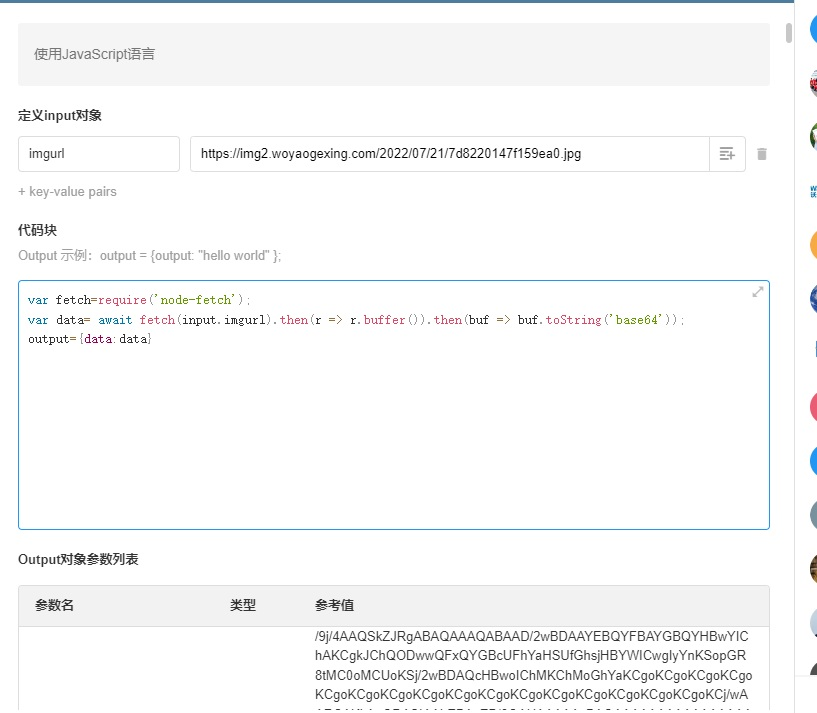var fetch=require(‘node-fetch’);

var data= await fetch(input.imgurl).then(r => r.buffer()).then(buf => buf.toString(‘base64’));

output={data:data}

……